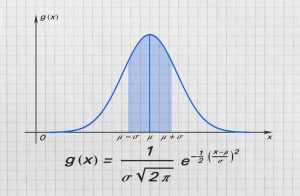## What math is required for statistics?

Statistics is a field that uses mathematical and computational methods to analyze data, model uncertainty, design experiments and surveys, and interpret empirical results. It is an interdisciplinary science that is important for all aspects of the natural and social sciences, particularly in the fields of biology, chemistry, ecology, economics, mathematics, neuroscience, sociology, psychology, and physics.The basic math required for statistics is not that difficult. It is the complexity of the formulas used to solve statistical problems and their application to specific situations that cause students to struggle.

This is especially true of statistics courses that deal with advanced methods of statistical analysis like time series and regression. These courses require a strong background in linear algebra and some calculus.

It is also necessary to be comfortable with algebra and calculus in order to properly understand the nuances of the equations being solved.

In addition, you need to have a solid understanding of probability theory and distributions. These concepts are integral to statistics and are taught in many of the courses required for a major in statistics.

Moreover, you should be familiar with some of the tools and programs used in statistics. Most courses require the use of software packages to help in the study of statistical techniques.

Some of the more complex calculations in statistics involve the use of matrices and functions which are all topics covered in linear algebra.

The math requirements for the major depend on the type of statistics you are interested in studying. You will need a strong background in linear algebra for most multivariate statistics courses and some knowledge of probability and distributions for more specialized courses.

You should be able to understand the concepts of probability and statistics in a way that allows you to explain the results of your analysis to people outside of your field. This is especially crucial if you plan on going to graduate school or working in the business world.

For instance, if you want to work in the health sciences you may be required to take a course in epidemiology or if you want to go into actuarial work you will likely need a degree in statistics.

In addition, you should be able to think critically about the statistics that you are learning and apply them in real-world situations. This will help you become a better student and eventually a better statistician.

You should be able to communicate your findings in a clear and concise manner, both in writing and orally. This is especially important if you are planning to go into the workforce and will need to present your results to non-technical audiences. You should also be able to work with and interpret data that is in a large variety of formats.

In conclusion, studying statistics requires a solid foundation in mathematics, particularly in algebra, calculus, linear algebra, probability theory, and distributions. While the basic math concepts themselves are not overly complex, it is the application of formulas and solving statistical problems that often poses challenges for students.

Advanced statistics courses, such as those focusing on time series and regression analysis, necessitate a strong background in linear algebra and some calculus. Understanding the nuances of equations and being comfortable with algebra and calculus are essential for comprehending and effectively applying statistical methodologies.

Probability theory and distributions are integral components of statistics, and a thorough understanding of these concepts is necessary. These topics are covered in many required courses for a statistics major.

Proficiency in statistical software packages and tools is also crucial, as these tools are commonly employed in statistical analysis. Additionally, complex calculations in statistics often involve matrices and functions, which are covered in linear algebra.

The specific math requirements for a statistics major may vary depending on the area of specialization. For instance, multivariate statistics courses typically require a strong background in linear algebra, while specialized courses may demand knowledge of probability and distributions.

Beyond mathematical skills, effective communication is essential for a statistician. Being able to explain statistical concepts and analysis results to individuals outside of the field is vital, particularly for those pursuing graduate studies or working in industries such as healthcare or actuarial sciences.

Furthermore, critical thinking and the ability to apply statistical concepts to real-world situations are key attributes of a successful statistician. The capacity to analyze and interpret data in various formats and present findings clearly and concisely, both in written and oral forms, is crucial for success in the field.

By developing a strong mathematical foundation, mastering statistical methodologies, and honing communication and critical thinking skills, students can become proficient statisticians capable of applying their knowledge in diverse contexts.